## Average

INTRODUCTION
The Concept of averages is important. Some exams test the concept of average through direct questions the entrance exams have used the concept to set Conceptual problems that ordinarily will be difficult to be tackled.
For that while studying this chapter make sure to understand the faster illustrated computation in the text.
Average =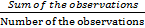Average Increase =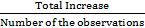Average Decrease =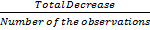SOLVED EXAMPLES
Example 1 Average of A, B, C & D = 45, Average of B, C, D & E = 68. If A = 100, Find E.
Solution: E = A + (Average of BCDE — Average of ABCD) x 4
E = 100 + (68 - 45) x 4
E = 100 + 23 x 4 = 192

Example 2 Average of A, B C & D = p Average of A & B = q, Average of C & D = ?
Solution: Average of CD =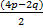= 2p - q
Example 3 Average Salary of 24 persons decreases by Rs. 50 when one of them drawing Rs.12200 retires and replaced by a new man. What is the salary of the new comer?
Solution: Salary of the new corner = Rs. (12200 - 24 x 50) = Rs. 11000
Example 4 Average of A, B, C & D = 43, Average of C, D, E & F = 53, Average of A & B = 30, Average of E & F = ?
Solution: Average of EF =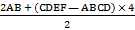Average of EF =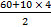= 50
Example 5 Average of A, B, C, D, E, F & G =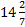, Average of A, B, C & D = 20. Average of E & F = 5, Find G.
Solution: G =x 7 – 20 x 4 - 5 x 2 = 10
Example 6 What is the average of first 37 odd numbers.
Solution: Sum of first n odd numbers is n2.Therefore, the average is n., Hence n = 37
Example 7 What is the average of first 38 even numbers.
Solution: Sum of first n even numbers is n(n +1)
Therefore, the average is n + 1, Hence, the average of first 38 even numbers is 39.
Example 8 Average of 0.1, x & 0.001 = 0,037, x = ?
Solution: x = 0.037 x 3 - 0.1 - 0.001 = 0.01
Example 9 Average of 20, 30 and x = 100, Average of 30, 40, x & y = 200, y = ?
Solution: x = 300 - (20 + 30) = 250
y = 800 – [30 + 40 + 250] = 480
Example 10 Average of ABCDE = 37, Average of BCDEF = 48, A : F = 2 : 13, A = ?
Solution: F - A = (48 - 37) x 5 = 55
A= 2 x 5 = 10
Example 11 Average of any 5 consecutive multiples of 28 is 56056. Find the smallest multiples out of those.
Solution: Smallest multiple of 28 = 56056 - 56
Smallest multiple of 28 = 56000
Example 12 A = 60, B = 60, C = 60, D is 60 more than average of A, B, C & D, then D = ?.
Solution: Let average of A, B, C & D be x
D = 60 + x
x =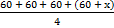4x = 240 + x
x = 80 D = 140
Example 13 A = 60, B = 60, C = 60, D is 30 less than average of A, B, C & D, D = ?
Solution: Let the average of A, B, C & D be x
D = x - 30
x =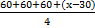4x = 150 + x
x = 50  D = 20
Example 14 Average of x, y & z = 100, (x - 20) ; (y + 80) = 2/3 , then z = ?
Solution: x + y + z = 300;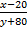= 2/3 i.e., 3 (x - 20) = 2 (y + 80)
3x - 60 = 2y + 160
3x - 2y = 220; 2x + 2y + 2z = 600  , 5x + 2z = 820
2z = 820 - 5x
Z =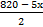…(i)
3x - 2y = 220  3x - 220 = 2y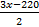= y …(ii)
x + y + z = 300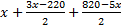= 300  x = 120
2z = 820 - 600  z = 110

Example 15 In Section A, 20 Students average weight is 40 kg. In Section B, 60 Students average weight is 60 kg. In Section C, 20 Students average weight is 70 kg. What will be the average of all the 100 students.
Solution: Average of all 100 students is =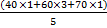= 58
Example 16 Average age of ABC 4 years ago was 13 years. D was born 9 years ago. What is the present average age of ABCD?
Solution: Average age of ABCD =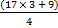= 15 years.
Example 17 Speed from A to B = 40 km/h, Speed from B to A = 60 km/h, Average speed = ?
Solution: Average speed =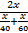= 48 km/h
Example 18 Average of ABCD = 40, Average of BCDE = 100, E = 300, A = ?
Solution: B + C + D = 100
A = 40 x 4 - 100 = 60
Example 19 Find the average of first 100 natural numbers.
Solution: Average of first 100 natural numbers =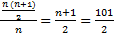= 50.5
Example 20 What is the increase in avg, if the 16th multiple of 26 is added to any 15 consecutive multiples of 26.
Solution: Increase in average = 26/2 = 13.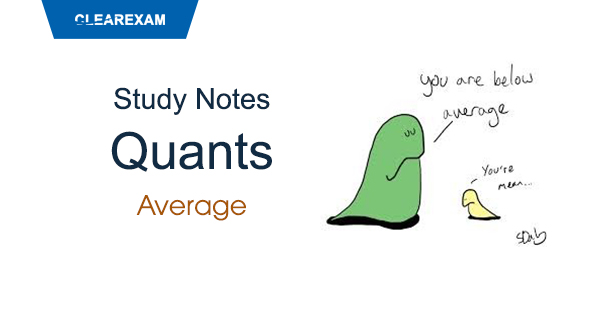Want to Know More
Please fill in the details below:

Name

ltr
item
Clear CTET - Coaching Institute for CTET, DSSSB and KVS: dsssb-quants-average-theory-part1
dsssb-quants-average-theory-part1